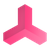0

# ABB Word mapping

Bo Adam 1 year ago updated 1 year ago

Hello,

A small time ago we had a conversation about adding bytes into the device mapping of ABB, now i needs words (16bits).

We are trying to get the Word option to work as well, we have changed the code to the following:

Only thing we are not sure about is the line with accessor.Write(posheader, (byte) 10).

Is this correct or should we change it?

Sorry for the formatting, couldn't find the code button.

if (signal.OriginDataType.ToUpper() == "BYTE")

{

if (signal.GetType() == typeof(PLCInputInt) || signal.GetType() == typeof(PLCOutputInt))

{

if (lastbit)

posmemory = posmemory + 1;

bit = 0;

shm.bit = 0;

lastbit = false;

signal.Comment = \$"M{posmemory}";

shm.type = SIGNALTYPE.BYTE;

posmemory = posmemory + 1;

}

}

else if (signal.OriginDataType.ToUpper() == "WORD")

{

if (signal.GetType() == typeof(PLCInputInt) || signal.GetType() == typeof(PLCOutputInt))

{

if (lastbit)

posmemory = posmemory + 1;

bit = 0;

shm.bit = 0;

lastbit = false;

signal.Comment = \$"M{posmemory}";

shm.type = SIGNALTYPE.WORD;

posmemory = posmemory + 2;

}

}

else

{ if (signal.GetType() == typeof(PLCInputInt) || signal.GetType() == typeof(PLCOutputInt))

{

if (lastbit)

posmemory = posmemory + 1;

bit = 0;

shm.bit = 0;

lastbit = false;

signal.Comment = \$"M{posmemory}";

shm.type = SIGNALTYPE.INT;

posmemory = posmemory + 4;

}

}

Sorry if you saw my answer on the last message, but i thought it would be good to make a new topic!+1